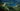# ES 提案：String.prototype.matchAll

## 使用正则表达式获取所有匹配

### RegExp.prototype.exec() 配合 `/g`

``````function collectGroup1(regExp, str) {
const matches = [];
while (true) {
const match = regExp.exec(str);
if (match === null) break;
// Add capture of group 1 to `matches`
matches.push(match);
}
return matches;
}

collectGroup1(/"([^"]*)"/gu, `"foo" and "bar" and "baz"`);
// [ 'foo', 'bar', 'baz' ]``````

``````let re = /[abc]/;
re.exec('abc');
// -> [ 'a', index: 0, input: 'abc' ]
re.exec('abc');
// -> [ 'a', index: 0, input: 'abc' ]``````

### String.prototype.match() 配合 `/g`

``````'abab'.match(/a/gu);
// -> [ 'a', 'a' ]``````

``````'abab'.match(/a/u);
// -> [ 'a', index: 0, input: 'abab' ]``````

### String.prototype.replace() 配合 `/g`

``````function collectGroup1(regExp, str) {
const matches = [];
function replacementFunc(all, first) {
matches.push(first);
}
str.replace(regExp, replacementFunc);
return matches;
}

collectGroup1(/"([^"]*)"/gu, `"foo" and "bar" and "baz"`);
// -> [ 'foo', 'bar', 'baz' ]``````

### RegExp.prototype.test()

`.test()` 只要正则表达式匹配就返回 `true`

``````const regExp = /a/gu;
const str = 'aa';
regExp.test(str); // true
regExp.test(str); // true
regExp.test(str); // false``````

### String.prototype.split()

``````const regExp = /<(-+)>/gu;
const str = 'a<--->b<->c';
str.split(regExp);
// -> [ 'a', '---', 'b', '-', 'c' ]``````

## 这些方法的问题

• 冗长且不直观
• 只有设置标志 `/g` 时才作用，有时我们会从其它地方收到正则表达式，例如通过参数。 然后如果我们要确保找到所有匹配项，则必须检查是否设置了此标志。
• 为了跟踪进度，所有方法（`.match()` 除外）都会更改正则表达式：`.lastIndex` 属性记录上一个匹配项的结束位置。 这使得在多个位置使用相同的正则表达式具有风险。尽管通常不建议这样做，但遗憾的是，当多次使用 `.exec` 时，不能内联正则表达式（因为每次调用都会重置正则表达式）：
``````// 没有用
const match = /abc/gu.exec(str);``````
• 由于属性 `.lastIndex` 确定匹配在何处继续，因此当我们开始收集匹配项时，该值必须始终为零。但是，至少 `.exec()` 在最后一次匹配之后将其重置为零。如果不为零，会发生以下情况：
``````const regExp = /a/gu;
regExp.lastIndex = 2;
regExp.exec('aabb'); // null``````

## String.prototype.matchAll() 提案

``const matchIterator = str.matchAll(regExp);``

``````[...'-a-a-a'.matchAll(/-(a)/gu)];
// -> [ [ '-a', 'a' ], [ '-a', 'a' ], [ '-a', 'a' ] ]``````

`.matchAll()` 不关心是否设置标志 `/g`

``````[...'-a-a-a'.matchAll(/-(a)/u)];
// -> [ [ '-a', 'a' ], [ '-a', 'a' ], [ '-a', 'a' ] ]``````

``````function collectGroup1(regExp, str) {
let results = [];
for (const match of str.matchAll(regExp)) {
results.push(match);
}
return results;
}``````

``````function collectGroup1(regExp, str) {
let arr = [...str.matchAll(regExp)];
return arr.map((x) => x);
}``````

``````function collectGroup1(regExp, str) {
return Array.from(str.matchAll(regExp), (x) => x);
}``````

### .matchAll() 返回一个不可重新开始的迭代器

`.matchAll()` 返回一个迭代器，并且不可重新开始。因此，一旦结果用尽，需要再次调用该方法并创建一个新的迭代器。

## 实现一个 .matchAll() 方法

``````function ensureFlag(flags, flag) {
return flags.includes(flag) ? flags : flags + flag;
}
function* matchAll(str, regex) {
const localCopy = new RegExp(regex, ensureFlag(regex.flags, 'g'));
let match;
while ((match = localCopy.exec(str))) {
yield match;
}
}``````

• `/g` 被设置。
• `regex.lastIndex` 没有改变。
• `localCopy.lastIndex` 是零。

``````const str = '"fee" "fi" "fo" "fum"';
const regex = /"([^"]*)"/;

for (const match of matchAll(str, regex)) {
console.log(match);
}

// 输出:
// fee
// fi
// fo
// fum``````